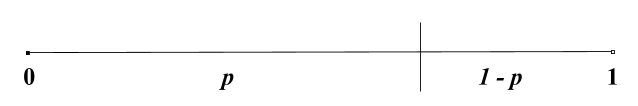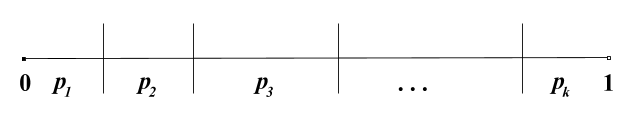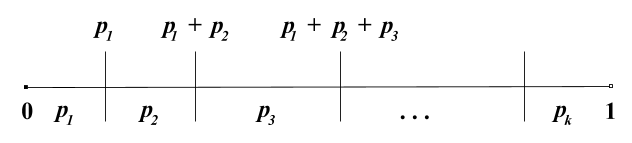# Building Binomial and Multinomial Samplers in Java

I recently came across the term multinomial sampler and was a bit embarassed that I’d never heard of it before. So, I decided to dig a bit deeper and implement one in Java. What follows is an overview of the topic.

A multinomial sampler samples from the multinomial distribution. I know, that’s a pretty useless definition unless you already know what it is. To understand a multinomial distribution it’s easier to first look at the binomial distribution, which is a special case of a multinomial distribution.

## The Binomial Distribution

The binomial distribution models, or captures the relevant details, of scenarios like the following:

1. You do a trial or experiment that yields two possible outcomes, e.g. a success or a failure. (Each experiment is independent and the probability of a success is always $$p$$.)
2. You repeat this trial $$n$$ times.
3. You want to know what number of successes (and hence the number of failures) to expect. (The combined number of successes and failures equals $$n$$)

The classic example is a coin flip. A single coin flip yields either heads (call this the “success”) or tails (call this the “failure”), and is our “experiment” above. In this example, a binomial distribution would help us answer questions like:

• If I flipped this coin 10 times, what is the probability I’d get exactly 5 heads?
• If I flipped this coin 10 times, what is the probability I’d get 5 or more heads?

Such a model is useful because we don’t have to spend all day flipping coins to determine what the probability might be based on long run frequencies; instead we can make certain assumptions and calculate the probability directly. It is applicable in any situation where there are only two possible outcomes, and each outcome is independent of all others.

## Sampling from a Binomial Distribution

We’ve talked about how the binomial distribution allows us to calculate the exact probability of a certain number of successes over n trials. But what if we want to sample from this distribution, i.e. to get the result of n trials? How can this simulation be done? Let’s go over how to build something like this, which is also known as a random variate generator.

For each single trial, the probability of success is $$p$$ and the probability of failure is $$1-p$$. If we have a way of getting a uniformly-distributed random variable (as is the case with most programming languages), we can transform this sample into the outcome of a single trial in the following manner:

1. Get a sample from a uniformly distributed random variable between $$[a, b)$$. For the sake of simplicity, assume that $$a = 0$$ and $$b = 1$$ so this is the standard uniform distribution.
2. Map a proportion of these values equal to $$p$$ to “success” and the remainder to “failure”.

This can be visualized on the following number line:The code for this is then straightforward:

// NOTE: Lacks input validation.
public boolean binomialTrial(final double p) {
// nextDouble() returns a uniformly-distributed value between 0.0 inclusive
// and 1.0 exclusive.
if (_random.nextDouble() < p) {
return true;
}
return false;
}


We can then build upon this to get a sample from a binomial distribution with parameters $$n$$ (number of trials) and $$p$$ (probability of succcess of each trial):

/**
* Returns a sample from a Binomial Distribution with parameters
* n (number of trials) and p (probability of success of an individual
* trial).
*
* The sample is the number of successes observed.
*/
public int sample(final int n, final double p) {
// Basic error checking.
if (n <= 0) {
return 0;
} else if (p >= 1) {
return n;
} else if (p <= 0) {
return 0;
}

int successes = 0;
for (int i = 0; i < n; i++) {
if (binomialTrial(p)) {
successes++;
}
}
return successes;
}


(The full code is available here)

### Making it more efficient

The downside of a straightforward/naive implementation like the one above is that the runtime of obtaining a sample scales linearly with the number of trials ($$n$$) that we parameterize the distribution with. The runtime efficiency here be improved in a number of ways that are outlined and summarized in the book, Non-Uniform Random Variate Generation (Devroye, 1986).

These methods are outlined on Page 523 in Chapter 10 of the book. Some involve generating a geometric random variable and using that to generate the binomial random variable in such a way that the runtime is reduced to $$O(np)$$ instead of $$O(n)$$. (An example of that is seen here)

For cases where $$n$$ is very large, a technique called Rejection Sampling can be used. In this case, a normal distribution is partially used to approximate the bounds. Devroye also details this approach (which he calls the “Rejection method”) on Page 533 of Chapter 10. This leads to a constant-time implementation, as the runtime does not depend on the value of $$n$$ or $$p$$, though the implementation is considerably more complicated.

Another way that trades time for space is to pre-compute the probabilities of each outcome. That is, for each value of $$k$$ (the number of successes) for a fixed value of $$n$$ (the number of trials), we’ll compute the probability of that outcome directly using the probability mass function (PMF) for the binomial distribution. Since $$k \in {0, 1, …, n}$$ this means we’ll have to store $$n + 1$$ probabilities. Then, to sample from the distribution, we can generate a single uniform random variable between $$[0, 1)$$ and map an appropriate proportion of the range to each outcome based on the pre-computed probabilities. This technique is probably suitable when $$n$$ is known/fixed ahead of time and you need to generate samples frequently.

## The Multinomial Distribution

The multinomial distribution an extension (or generalization) of the binomial distribution where instead of two distinct outcomes of each trial, there are instead $$k$$ mutually exclusive outcomes. In other words, the binomial distribution is a special case of the multinomial distribution where $$k = 2$$.

In this case, we are counting the number of each of the $$k$$ outcomes that happen after we do $$n$$ independent experiments or trials, where each outcome $$k$$ has an associated probability for a single trial.

An example of this would be rolling two dice and totaling the values. In this experiment, there are 11 possible outcomes (the values 2-12 inclusive) each of which have an associated probability. We could then use the multinomial distribution to calculate what the probability would be of getting exactly a certain number of each value after doing $$n$$ rolls of the dice.

Note that in the general case, a multinomial distribution is a multivariate distribution, because describing the outcome requires more than one value; for $$k$$ possible outcomes, you usually need a vector $$X$$ of length $$k$$, with component $$X_i$$ describing the number of outcomes of $$i$$ over the $$n$$ trials.

## Sampling from a Multinomial Distribution

To sample from a multinomial distribution, we first have to build a way to get the result from a single multinomial trial. That is, given $$k$$ possible outcomes with probabilities $$p_1, p_2, … p_k$$ (all of which sum to 1), for a single trial we need to randomly select one of these outcomes according to their probabilities.

As with a binomial trial, we can accomplish this by using the output of a uniform random variable between $$[0, 1)$$. Visualizing this with a number line is helpful again:To convert the output from a uniform random variable between $$[0, 1)$$ we have to map a proportion of the values to each outcome where the proportion of values mapped to each outcome is determined by the probability $$p_i$$ of that outcome. For example, if a certain outcome has a probability of 0.3, then 30% of the range of values between $$[0, 1)$$ should map to this outcome. In other words, each outcome will map to a certain range of values from a uniform random variable.

This becomes easier to implement if we think of the boundaries of each range from the number line above. For example, the first outcome has an upper boundary of $$p_1$$, the second outcome has an upper boundary of $$p_1 + p_2$$, the third has an upper boundary of $$p_1 + p_2 + p_3$$ and so on. The final outcome $$k$$ will always have an upper boundary of $$1$$ by definition. Our number line then looks like this:When visualized like this, the problem of finding which range or bin that the output of a uniform random variable maps to is reduced to this:

1. Generate a uniform random variable between $$[0, 1)$$.
2. Going through the bins one by one and starting from the left, pick the first bin whose upper boundary is greater than the uniformly-sampled value.
3. The index of the bin selected corresponds to the outcome of a single multinomial trial.

This results in code like this:

/**
* The _probabilities field is an array of "cumulative" probabilities.
* The first element has the value p1, the second has p1 + p2, the third has p1 + p2 + p3, etc.
* By definition, the last bin should have a value of 1.0.
*/
public int multinomialTrial() {
double sample = _random.nextDouble(); // Between [0, 1)
for (int i = 0; i < _probabilities.length; ++i) {
// Find the first bucket whose upper bound is above the sampled value.
if (sample < _probabilities[i]) {
return i;
}
}
// Catch-all return statement to ensure code compiles.
return _probabilities.length - 1;
}


(This is an implementation of the procedure described here, though it does not include the optimization to sort the probabilities in descending order.)

Then, to get a sample from a multinomial distribution representing $$n$$ trials, we just have to call the above function $$n$$ times and record the counts for each outcome to an array that is a random vector representing the sample:

/**
* Returns the result of a multinomial sample of n trials.
* The value of k (the number of possible outcomes) is determined by the
* number of probabilities passed into the constructor.
*/
public int[] multinomialSample(final int n) {
// The length of _probabilities is the number of possible outcomes.
final int result[] = new int[_probabilities.length];

// Get the result of each trial and increment the count for that outcome.
for (int i = 0; i < n; i++) {
result[multinomialTrial()]++;
}

return result;
}


(The full code is available here)

### Making it more efficient

When I first thought about improving the efficiency, I was focused on making the single trial faster; instead of doing a linear scan through all the ranges, I thought about using a binary search tree (BST) to speed up the lookup. However, the degree of improvement this approach could offer depends on the individual probabilities of each outcome in the multinomial distribution.

If all probabilities are roughly equal, using something like a BST will help improve the lookup time to find which range the uniform random variable falls into, especially with a large value of $$k$$, i.e. the number of possible outcomes. However, if one of the probabilities is much higher than the other, then the simple approach described above, combined with sorting the probabilities in descending order (as detailed here) should work fairly well.

Another approach, also outlined by Devroye (on page 558 of Chapter 11; also check out the errata) focuses on optimizing the process of getting the sample (of $$n$$ trials) versus trying to optimize the single trial. It works based on this recurrence relation:

Assume that $$X$$ is a random vector from the multinomial distribution with parameters $$n$$ (number of trials) and $$k$$ possible outcomes for each trial, with probabilities $$(p_1, …, p_k)$$. Then $$X$$ is a random vector in $$\mathbb{Z}^k$$ with each component being non-negative.

Then $$X_1$$ (the first component) is from a binomial distribution with parameters $$n$$ and $$p_1$$. The remaining components form a vector $$\langle X_2, …, X_k \rangle$$ which is from a multinomial distribution with parameters $$n - X_1$$ and probabilities $$(q_2, …, q_k)$$ where $$q_j = {p_j / {(1 - p_1)}}$$.

We can repeat this process $$k$$ times to get each component of $$X$$ by sampling from an appropriate binomial distribution.

Using this recurrence relation, we can generate a multinomial random vector component-wise, which results in the random vector being built by sampling from a binomial distribution up to $$k$$ times, once for each component of the random vector. Sample code for this implementation is below:

/**
* Returns the result of a multinomial sample of n trials.
* The value of k (the number of possible outcomes) is determined by the
* number of probabilities passed in.
* <p>
* This assumes that probabilities sums to 1.0.
*/
public int[] multinomialSample(final int n, final double[] probabilities) {
// Basic error checking:
if (n <= 0 || probabilities == null || probabilities.length == 0) {
return new int;
}

final int[] result = new int[probabilities.length];
double remainingSum = 1.0;
int remainingTrials = n;
for (int i = 0; i < probabilities.length; i++) {
// _binomialSampler returns a sample from a binomial distribution (n, p)
result[i] = _binomialSampler.sample(remainingTrials, probabilities[i] / remainingSum);

remainingSum -= probabilities[i];
remainingTrials -= result[i];

// Due to floating-point, even if the probabilities appear to sum to 1.0 the remainingSum
// may go ever so slightly negative on the last iteration. In this case, remainingTrials will
// also be 0. (But remainingTrials may go to 0 before the last possible iteration)
if (remainingSum <= 0 || remainingTrials == 0) {
break;
}
}
return result;
}


(The full code is available here)

The efficiency of this implementation hinges on the efficiency of the binomial sampler it uses. For very large values of $$n$$, again using a rejection sampling approach for sampling from the binomial distribution is most efficient. However, for smaller values of $$n$$ and $$k$$ using a straightforward binomial sampler is probably sufficient.

If we just use a straightforward implementation of a binomial sampler, then the multinomial sampler based on the recurrence relation isn’t any more efficient. A (very basic, perhaps limited) JMH test shows this:

Benchmark                                              Mode  Cnt   Score   Error  Units
MultinomialBenchmark.testMultinomialRecurrenceSampler  avgt    5  10.773 ± 0.138  ms/op
MultinomialBenchmark.testSimpleMultinomialSampler      avgt    5  10.948 ± 0.366  ms/op


(Code used to generate the JMH test is available here)

## Conclusion

I’ve provided an introduction to building a binomial sampler as well as a multinomial sampler, and then given a brief overview on how each might be made more efficient from a runtime perspective. However, we shouldn’t miss the forest for the trees. Unless your application is doing something very specific that’s tied to a huge amount of random sampling, it’s unlikely that optimizing this would result in a meaningful improvement, and hence the added code complexity may not be worthwhile. As always, you should measure and profile your code to determine which areas should be targeted for optimization in order to yield the biggest benefit.

Additionally, testing any sort of random algorithm for correctness is going to be more tricky than doing the same for a deterministic one. You should subject the output of any random generator to some sort of statistical hypothesis test to see if the difference between the output distribution and the expected distribution is statistically significant - in which case the generator likely has a flaw that is introducing unwanted bias. (This includes the sample code I’ve provided!) Because of this, all else being equal, you should prefer a simpler implementation than a more complicated one.

Hopefully this article has served as starting point to pique your interest on random variate generators. If you’re interested, the previously mentioned book, Non-Uniform Random Variate Generation (Devroye, 1986) is a good starting point that begins with the fundamentals and covers a wide variety of techniques for building random variables from various distributions. (The author has also chosen to make the book freely available) I think it’s a good starting point before delving into any more recent literature.